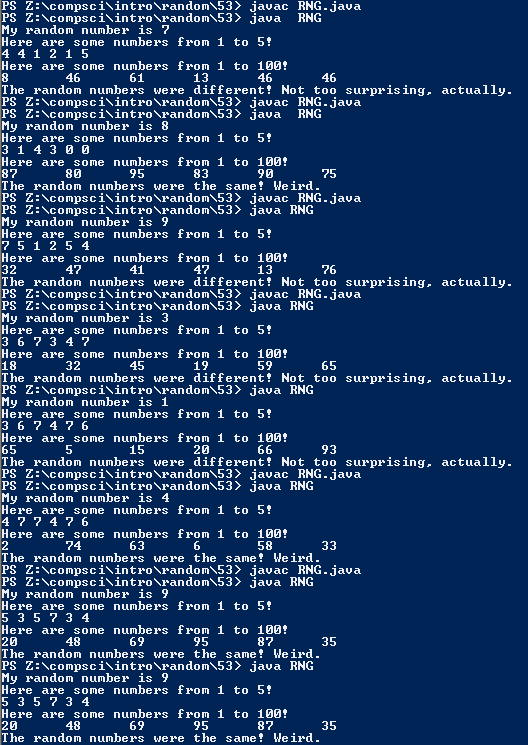# Assignemnt #53

## Code

```///colin hinton
///5th period
///RNG
///RNG.java
///10/28/2015

import java.util.Random;

public class RNG
{
public static void main ( String[] args )
{
Random r = new Random();
// Whatever the seed is determins what the random numbers will be, but with seed 2 for instance they will always be the same.

int x = 1 + r.nextInt(10);

System.out.println( "My random number is " + x );

System.out.println( "Here are some numbers from 1 to 5!" );
System.out.print(  3+ r.nextInt(5) + " " );
System.out.print(  3+ r.nextInt(5) + " " );
System.out.print(  3+ r.nextInt(5) + " " );
System.out.print(  3+ r.nextInt(5) + " " );
System.out.print(  3+ r.nextInt(5) + " " );
System.out.print(  3+ r.nextInt(5) + " " );
System.out.println();
// By removing the (1 +) since 1 was the starting number or lowest, zero is now an answer.
// By adding different numbers before the r.nextInt(), it breaks the range that was there before. The range is now 3-7, weird.
System.out.println( "Here are some numbers from 1 to 100!" );
System.out.print( 1 + r.nextInt(100) + "\t" );
System.out.print( 1 + r.nextInt(100) + "\t" );
System.out.print( 1 + r.nextInt(100) + "\t" );
System.out.print( 1 + r.nextInt(100) + "\t" );
System.out.print( 1 + r.nextInt(100) + "\t" );
System.out.print( 1 + r.nextInt(100) + "\t" );
System.out.println();

int num1 = 1 + r.nextInt(10);
int num2 = 1 + r.nextInt(10);

if ( num1 == num2 )
{
System.out.println( "The random numbers were the same! Weird." );
}
if ( num1 != num2 )
{
System.out.println( "The random numbers were different! Not too surprising, actually." );
}
}
}

```

### Picture of the output